DOC HOME SITE MAP MAN PAGES GNU INFO SEARCH PRINT BOOK
Floating point operations

# Normalized numbers

A number is normalized if the exponent field contains other than all 1's or all 0's.

The exponent field contains a biased exponent, where the bias is 127 in single-precision, 1023 in double-precision, and 16,383 in extended-precision. Thus, the exponent of a normalized floating point number is in the range -126 to 127 inclusive for single-precision, and in the range -1022 to 1023 inclusive for double-precision. For extended-precision the range is -16,382 to 16,383.

There is an implicit bit associated with both single- and double-precision formats. The implicit bit is not explicitly stored anywhere (thus its name). Logically, for normalized operands the implicit bit has a value of 1 and resides immediately to the left of the binary point (in the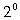position). Thus the implicit bit and fraction field together can represent values in the range 1 to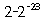inclusive for single-precision, and in the range 1 to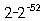inclusive for double-precision. For extended-precision, there is no such bit, therefore the field can represent values in the range 1 to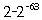Thus normalized single-precision numbers can be in the range (plus or minus)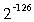to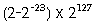inclusive.

Normalized double-precision numbers can be in the range (plus or minus)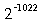to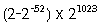inclusive.

Normalized extended-precision numbers can be in the range (plus or minus)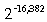to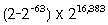inclusive.

Next topic: Denormalized numbers
Previous topic: Extended-precision